# Wildcard matching — Dynamic Programming made easy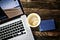Photo by Emily Finch on Unsplash

In this post, through the wildcard matching problem, I want to highlight the fact that dynamic programming is very intuitive, and once you are familiar with recursion, dynamic programming will add to it a little idea, but this idea improves the performance of programs drastically.

I think one of the most important questions about Dynamic Programming is how do we spot that the problem is a DP problem. …

# Drawing random trees using geometry and recursion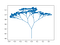A Tree generated using the program described below

The program used to generate such trees is a simple but beautiful mixture of algorithms’ recursion and simple geometry. The code is available on github and I provide here some explanation of how it works.

The algorithm is a simple recursive approach: From each branch we are going to generate two child branches until we reach some depth d. A branch here is a segment defined by its extremity points.

The child branches will have a different angle from the parent branch. To make the generated tree more realistic it’s better if one branch has an angle smaller than the…

# System call — How it works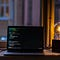Photo by Oskar Yildiz on Unsplash

A system call can be defined as a request made to the OS so that it executes some operations for the user process. In modern operating systems, some operations can’t by any means be performed directly by the user process without the help of the OS. Examples of such operations are a read from disk, a write to disk, a fork of the process, etc…

## Why do we need system calls

There are two main reasons why such instructions are not available directly for the process. The first one is protection: The OS must do many checks to evaluate the right of the process to request…

# Area of Rectangle equals Length x Width — Proof ?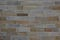Photo by Edouard Dognin on Unsplash

This is indeed a very basic question. But trying to answer it can teach some important things, and perhaps help see how mathematics is built.

In the first section, I try to give several motivations why this is an important question. If you are already aware of that you can skip it and jump directly to the section Looking for a proof”.

# Why is this an important question

Something that is widely used in mathematics is the formula:

Area of rectangle = Length x Width

This is one of the most used formulas in Geometry. For example, whenever we deal with the area of a triangle…

# Longest Well Performing Interval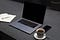Photo by Unsplash on Unsplash

In this post, I share some classical ways to optimize Brute force solutions for array problems through Longest Well-Performing Interval.

The problem is the following:

We are given `hours`, a list of the number of hours worked per day for a given employee.

A day is considered to be a tiring day if and only if the number of hours worked is (strictly) greater than `8`.

A well-performing interval is an interval of days for which the number of tiring days is strictly larger than the number of non-tiring days.

Return the length of the longest well-performing interval.

Example:

`Input…`

# 132 Pattern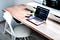Photo by Alexandru Acea on Unsplash

In this post, I would like to give the detailed thinking process that can lead you to the one pass O(N) stack solution.

It may be a little bit detailed as a post, but this is how we can come up with a solution. For problems like this one, we need to examine different test cases and think of a generalized algorithm that takes them all into account. This is programming in fact.

Problem:

Given a sequence of n integers a1, a2, …, an, a 132 pattern is a subsequence ai, aj, ak such that i < j < k

# Kth Smallest Element in Sorted Matrix

In this post, I would like to share my thinking process for this problem.

Problem:

Given a n x n matrix where each of the rows and columns are sorted in ascending order, find the kth smallest element in the matrix.

Example:

`matrix = [   [ 1,  5,  9],   [10, 11, 13],   [12, 13, 15]],k = 8,return 13.`

Source : Leetcode 378

Let’s take some examples to understand better the problem.

`# example 1 : any number from row i+1 is higher than anyone from row i. …`## Ayoub Omari

Computer Science, Physics & Maths Enthusiast